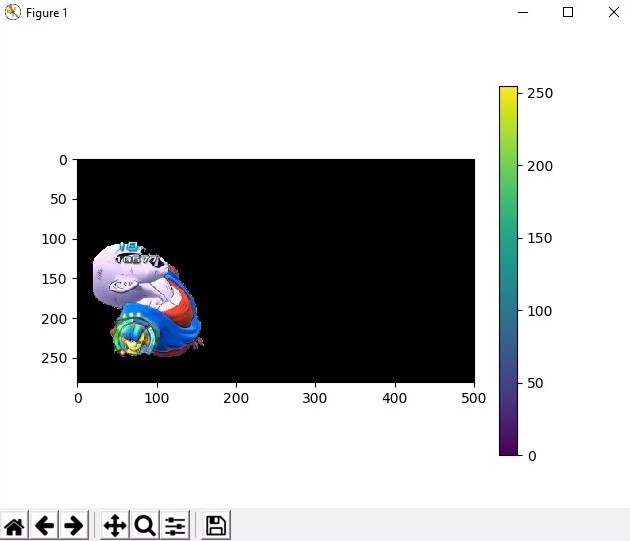# Python | Foreground Extraction in an Image using Grabcut Algorithm

• Difficulty Level : Expert
• Last Updated : 05 Jan, 2022

Let’s discuss an efficient method of foreground extraction from the background in an image. The idea here is to find the foreground, and remove the background.
Foreground extract is any technique which allows an image’s foreground to be extracted for further processing like object recognition, tracking etc. The algorithm used for foreground extraction here is GrabCut Algorithm. In this algorithm, the region is drawn in accordance with the foreground, a rectangle is drawn over it. This is the rectangle that encases our main object. The region coordinates are decided over understanding the foreground mask. But this segmentation is not perfect, as it may have marked some foreground region as background and vice versa. This problem can be avoided manually. This foreground extraction technique functions just like a green screen in cinematics.

• The region of interest is decided by the amount of segmentation of foreground and background is to be performed and is chosen by the user. Everything outside the ROI is considered as background and turned black. The elements inside the ROI is still unknown.
• Then Gaussian Mixture Model(GMM) is used for modeling the foreground and the background. Then, in accordance with the data provided by the user, the GMM learns and creates labels for the unknown pixels and each pixel is clustered in terms of color statistics.
• A graph is generated from this pixel distribution where the pixels are considered as nodes and two additional nodes are added that is the Source node and Sink node. All the foreground pixels are connected to the Source node and every Background pixel is connected to the Sink node. The weights of edges connecting pixels to the Source node and to the End node are defined by the probability of a pixel being in the foreground or in the background.
• If huge dissimilarity is found in pixel color, the low weight is assigned to that edge. Then the algorithm is applied to segment the graph. The algorithm segments the graph into two, separating the source node and the sink node with the help of a cost function which is the sum of all weights of the edges that are segmented.
• After the segmentation, the pixels that are connected to the Source node is labeled as foreground and those pixels which are connected to the Sink node is labeled as background. This process is done for multiple iterations as specified by the user. This gives us the extracted foreground.

The function used here is cv2.grabCut()

Syntax: cv2.grabCut(image, mask, rectangle, backgroundModel, foregroundModel, iterationCount[, mode])
Parameters:

• image: Input 8-bit 3-channel image.
• mask: Input/output 8-bit single-channel mask. The mask is initialized by the function when mode is set to GC_INIT_WITH_RECT. Its elements may have one of following values:
• GC_BGD defines an obvious background pixels.
• GC_FGD defines an obvious foreground (object) pixel.
• GC_PR_BGD defines a possible background pixel.
• GC_PR_FGD defines a possible foreground pixel.
• rectangle: It is the region of interest containing a segmented object. The pixels outside of the ROI are marked as obvious background. The parameter is only used when mode==GC_INIT_WITH_RECT.
• backgroundModel: Temporary array for the background model.
• foregroundModel: Temporary array for the foreground model.
• iterationCount: Number of iterations the algorithm should make before returning the result. Note that the result can be refined with further calls with mode==GC_INIT_WITH_MASK or mode==GC_EVAL.
• mode: It defines the Operation mode. It can be one of the following:
• GC_INIT_WITH_RECT: The function initializes the state and the mask using the provided rectangle. After that it runs iterCount iterations of the algorithm.
• GC_INIT_WITH_MASK: The function initializes the state using the provided mask. Note that GC_INIT_WITH_RECT and GC_INIT_WITH_MASK can be combined. Then, all the pixels outside of the ROI are automatically initialized with GC_BGD.
• GC_EVAL: The value means that the algorithm should just resume.

Below is the implementation:

## Python3

 `# Python program to illustrate``# foreground extraction using``# GrabCut algorithm`` ` `# organize imports``import` `numpy as np``import` `cv2``from` `matplotlib ``import` `pyplot as plt`` ` `# path to input image specified and``# image is loaded with imread command``image ``=` `cv2.imread(``'image.jpg'``)`` ` `# create a simple mask image similar``# to the loaded image, with the``# shape and return type``mask ``=` `np.zeros(image.shape[:``2``], np.uint8)`` ` `# specify the background and foreground model``# using numpy the array is constructed of 1 row``# and 65 columns, and all array elements are 0``# Data type for the array is np.float64 (default)``backgroundModel ``=` `np.zeros((``1``, ``65``), np.float64)``foregroundModel ``=` `np.zeros((``1``, ``65``), np.float64)`` ` `# define the Region of Interest (ROI)``# as the coordinates of the rectangle``# where the values are entered as``# (startingPoint_x, startingPoint_y, width, height)``# these coordinates are according to the input image``# it may vary for different images``rectangle ``=` `(``20``, ``100``, ``150``, ``150``)`` ` `# apply the grabcut algorithm with appropriate``# values as parameters, number of iterations = 3``# cv2.GC_INIT_WITH_RECT is used because``# of the rectangle mode is used``cv2.grabCut(image, mask, rectangle, ``            ``backgroundModel, foregroundModel,``            ``3``, cv2.GC_INIT_WITH_RECT)`` ` `# In the new mask image, pixels will``# be marked with four flags``# four flags denote the background / foreground``# mask is changed, all the 0 and 2 pixels``# are converted to the background``# mask is changed, all the 1 and 3 pixels``# are now the part of the foreground``# the return type is also mentioned,``# this gives us the final mask``mask2 ``=` `np.where((mask ``=``=` `2``)|(mask ``=``=` `0``), ``0``, ``1``).astype(``'uint8'``)`` ` `# The final mask is multiplied with``# the input image to give the segmented image.``image ``=` `image ``*` `mask2[:, :, np.newaxis]`` ` `# output segmented image with colorbar``plt.imshow(image)``plt.colorbar()``plt.show()`

Input Image:Output:Here we have taken an input image of size 500X281 and decided the coordinates for rectangle accordingly. The output image shows how the object in the left of the image becomes the part of the foreground and the background is subtracted.

My Personal Notes arrow_drop_up